# Exercise Module 9: Interactions

## Instructions:

This module accompanies the lectures from week 9.

This document contains information, questions, R code, and plots.

Hints and reminders are in bold

Questions appear in blue.

# R code looks like this.
# Comments are in grey and explain the code

2+2 # add two and two

##  4

# outputs from R appear like the above ^^^^


## Contents of module

• Part A = Choosing a model
• Part B = Two categorical explanatory variables
• Part C = Interactions
• Part D = Mixed continuous and categorical variables
• Part E = Detective skills
• Part F = Practice

## R skills

• Using lm() and interpretting output
• Plotting lm() results

## Part A: Choosing a model

If you want a recap on variables and data types then go here: https://qmbio.math.ntnu.no/theory/all-about-data/

If you fit a linear model to following datasets what would you be asking each time?

Dataset 1: Data collected on wing length of butterflies from three different populations; one at sea level, one half-way up a mountain, and one at the top. Wing length is in mm, the populations are “low”, “mid”, and “high” altitude. There are 100 measurements per population.

Dataset 2: Data collected on body weight and length of frogs. Body weight is in grams and length in mm. There are 200 measurements.

Dataset 3: Data collected on bacteria growth rate under two different treatments. The first treatment controls food and is “low” and “high”. The second concerns the presence of a fungus and is “present” or “not”. There is also a control for each treatment (treatments are food and fungus). There are 70 observations per treatment so 420 in total.

How does R find estimates of the parameters (the coefficients)?

Write tips to your future self on how to decide which model to use when.

## Part B: Two categorical explanatory variables

Last week you had data from an experiment carried out at Rothamsted research lab in the UK.

# Import the data

# Make sure to relevel the time variable
Yields$After1970 <- relevel(Yields$After1970, ref="Before") # Make before the intercept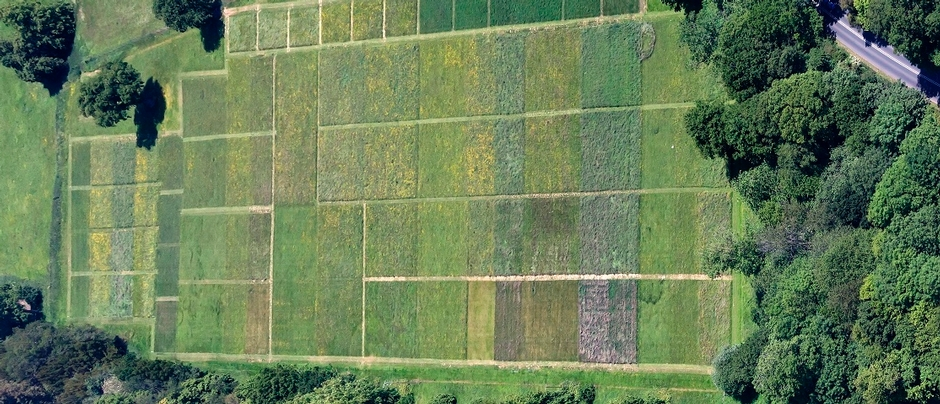The data had four fertiliser treatments: control, manure, fertilised, stopped. You analysed the effect of these different treatments last week using t-tests, linear models, and one-way ANOVA ideas.

Towards the end of last week Bob told you that in 1970 something changed in the experiment. We don't know exactly what changed, but we want to account for it. So, we introduced an extra variable:

After1970: with levels “Before” and “After”.

Run a model with both variables in.

Look at the output, what do the coefficients mean? Which parameters in the linear equation do they relate to?

$y_i = \alpha + \beta x_i + \varepsilon_i$

# Run the model
modelBoth <- lm(yield ~ Treatment+After1970, data = Yields)

# Look at coefficient estimates
coef(modelBoth)

##         (Intercept) TreatmentFertilised     TreatmentManure    TreatmentStopped      After1970After
##           0.6110417           1.9616667           3.0122222           0.8094444           0.9035417

# Look at uncertainty
confint(modelBoth)

##                         2.5 %    97.5 %
## (Intercept)         0.2525636 0.9695197
## TreatmentFertilised 1.4836959 2.4396374
## TreatmentManure     2.5342515 3.4901930
## TreatmentStopped    0.3314737 1.2874152
## After1970After      0.5450636 1.2620197


The code to run the model and print the estimates, with uncertainty, is shown above.

The interpretation of the coefficients in terms of the linear equation is as follows:

(Intercept) = $$\alpha$$ the $$y$$ value when $$x$$ = 0. In this case, $$x$$ doesn't actually have numeric values, instead they are groups. These can be coded as dummy variables, which do have numeric values of 0 for one group and 1 for the other. In this case $$x$$ = 0 = the control treatment group Before 1970. So, when $$x$$ = that group then the $$y$$ value = 0.611 = mean of this first group.

TreatmentFertilised = $$\beta_1$$ the slope of the effect of Fertilised. Any $$\beta$$ value tells us the amount of change in $$y$$ for a change of 1 in $$x$$. In this case, a change of 1 in $$x$$ = movement of one group. This value captures the change from the first group to the Fertilised group (still Before 1970). It is a difference between the two group means. If you add this estimate to the Intercept value you should get the mean of the Fertiliser group before 1970.

TreatmentManure = $$\beta_2$$ the slope of the effect of Manure. This is the same as $$\beta_1$$ but for the Manure group. It is still relative to the Intercept group (still Before 1970). If you add this estimate to the Intercept value you should get the mean of the Manure group before 1970.

TreatmentStopped = $$\beta_3$$ the slope of the effect of Stopped treatment. This is the same as $$\beta_1$$ but for the Stopped group. It is still relative to the Intercept group (still Before 1970). If you add this estimate to the Intercept value you should get the mean of the Stopped group before 1970.

After1970After = $$\beta_4$$ the slope of the effect of time After 1970. This is the same as $$\beta_1$$ but for the After1970 group, this is for the second variable. It is still relative to the Intercept group and the treatment level will be control. If you add this estimate to the Intercept value you should get the mean of the Control group after 1970.

The uncertainty numbers are for each of the effects listed about. So, the intercept row is uncertainty in a mean, but the rest are uncertainty of differences.

To find the mean of the different Treatments, After 1970, you would need to add the estimate of the Treatment effect to the estimate of the effect of time and add both to the Intercept.

Now you know what the coefficients should mean, check them by calculating the mean of each group in your data.

Code hint.

The code below shows you how you can easily (easy in terms of less typing) take the mean of each group in the data. Remember that [] are index brackets, they tell R, “I want something inside the object I just mentioned” e.g. object takes the first entry in object.

The == means matching and selects only entries that match what follows the ==. So, in words mean(Yields$yield[Yields$Treatment == "Control" & Yields\$After1970 == "Before"]) tells R to take the mean of the column yields inside data frame Yields but only for rows where column Treatment = Control and column After1970 = Before.

Why does it not add up?

## Part C: Interactions

Often in nature, effects are not separate but interact. This means that the effect of one variable is different for different values of another, e.g. plants might not be able to grow more in response to fertiliser, unless they also have enough light. So, the effect of fertiliser would be different in low and high light.

Often, we want to include interactions in our models. We do this by changing the + in lm() to * or :. If you use : need to use + too, * does everything. So we recommend using *! It's much easier. e.g. lm(Yield ~ Treatment * After 1970, data = Yields)

By adding in an interaction, we now change what R calculates from the model. In the previous example, without an interaction, the output of the lm() had no row for the Fertilised, Manure, or Stopped treatments for the time period After 1970. To work out what the estimated means of these groups you would have had to add the effect of the fertiliser treatment to the control and then add the effect of time after 1970. This assumes that the effect of time is the same for each fertiliser treatment AND that the effect of each fertiliser treatment is the same regardless of time.

But in reality, the effect of time might not be the same for each fertiliser treatment and vice versa, this is an interaction. So, when you fit a model with an interaction in R it fits a separate effect for each combination of groups within the interacting variables e.g. one for fertiliser after 1970 and a separate effect for fertiliser before 1970 and a separate one for control after 1970 etc.

When you run a model with an interaction, you should now get a row for each combination of time and fertiliser treatment.

Run the interactive model.

What do these coefficients mean? Which parameters do they relate to?

The (Intercept) row is still the mean of the control group before 1970. Each row that is labelled with 'Treatment' followed by a group name e.g. 'TreatmentFertilised' (but without a :) represent the difference in mean between the (Intercept) group and the named group (all before 1970). E.g. Fertilised before 1970 group has a mean 1.6225 more than the Control group before 1970.

The row that is labelled with After1970After gives the difference in mean between the (Intercept) group and the Control group after 1970.

The last few numbers are the interactions. They all incluce a : which tells you they are an interaction. They represent the difference between the mean of the Treatment group before 1970 and the mean of the Treatment group after 1970. For example, the row labelled TreatmentManure:After1970After represents the difference between the mean for the Manure treatment before 1970 (0.89750000 + 2.14083333) and the mean for the Manure treatment after 1970. After 1970, the Manure Treatment had a mean yield approximately 2.6 higher than before 1970.

# Run the model
modelBothI <- lm(yield ~ Treatment*After1970, data = Yields)

# Look at coefficient estimates
coef(modelBothI)

##                        (Intercept)                TreatmentFertilised
##                         0.89750000                         1.62250000
##                    TreatmentManure                   TreatmentStopped
##                         2.14083333                         0.87416667
##                     After1970After TreatmentFertilised:After1970After
##                         0.04416667                         1.01750000
##     TreatmentManure:After1970After    TreatmentStopped:After1970After
##                         2.61416667                        -0.19416667

# Look at uncertainty
confint(modelBothI)

##                                         2.5 %    97.5 %
## (Intercept)                         0.6204900 1.1745100
## TreatmentFertilised                 1.2307487 2.0142513
## TreatmentManure                     1.7490820 2.5325847
## TreatmentStopped                    0.4824153 1.2659180
## After1970After                     -0.4356288 0.5239621
## TreatmentFertilised:After1970After  0.3389668 1.6960332
## TreatmentManure:After1970After      1.9356334 3.2926999
## TreatmentStopped:After1970After    -0.8726999 0.4843666


Do things add up now? (we are not aiming for perfection)

What can you conclude from these results?

## Part D: Mixed continuous and categorical variables

What about continuous data? So far, treated both separately but there are many scenarios where we would want to have both types of explanatory variable in a single analysis.

Example 1: Body length and temperature and water treatment. Scientists measured the body length of insects in micrometers at different temperatures either with or without rainfall.

Example 2: Lay date and insect biomass and habitat. Scientists (including Emily) measured the date in the year when birds lay their eggs (it can take any value even 1.5 or -2) in different habitats in a woodland: good, bad, ok and also measured the biomass of insects close to each nest box. Biomass is measured in grams and there are several nest boxes in each habitat.

Example 3: Tail length and dinosaur family and age of fossil. Palentologists measured the tail length of fossils in cm and recorded the family the dinosaur belonged too and the age of the fossil in million years.

In the examples, which variable is categorical and which is continuous?

The data for example 1 (made up) can be found here: https://www.math.ntnu.no/emner/ST2304/2020v/Week09/BodyLengthExperiment.csv

It is a csv file and has column headers.

Run an interactive model for example 1.

What do these coefficients mean? Which parameters do they relate to?

Try and draw out the results on your whiteboard.

When drawing think about what data you have and how you can best represent it in a graph. Think about what the response variable is, what the explanatory variables are and how you would like to model them e.g. a straight line or several? Or differences in means etc. Don't worry, we will go through this afterwards so it doesn't matter if you are wrong, just have a go!

PAUSE HERE

Correct your drawing of the results if you need to.

Interpret output in biological terms.

Was an interaction term needed in this model?

# Part E: Detective skills

Look at the model outputs above, match the following model types to the output:

They are all in different styles and I have deliberately given no information on the type of data. You have to work it out from the output.

Example 1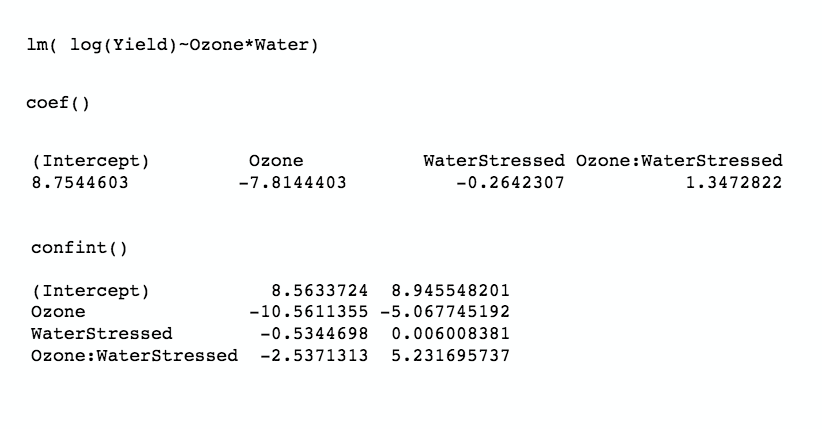Example 2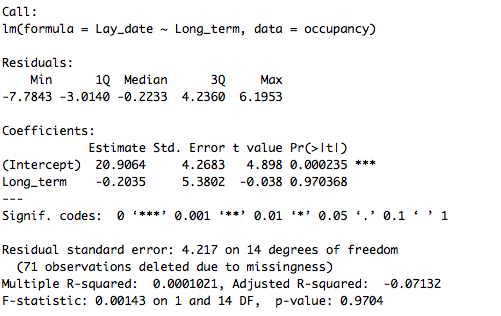Example 3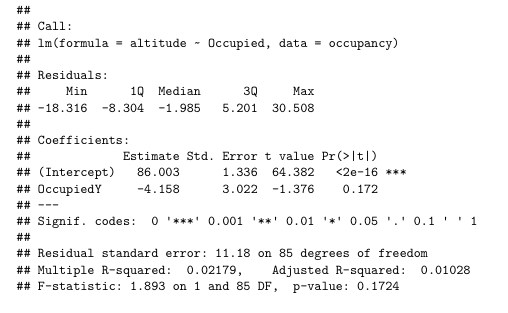Example 4: lm(time ~ mean_max_temp1*April_lay)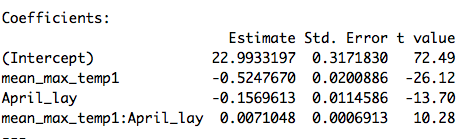• One categorical explanatory variable
• One categorical and one continuous explanatory variable
• One continuous explanatory variables
• Two continuous explanatory variables
• Model with an interaction

How would interpretation be different for a) a model with an interaction and the one with one categorical and one continuous explanatory variable b) the same data but no interaction?

## Part F: Practice -TOMORROW

Choose a dataset from the list below to analyse today.

Dataset 1: Cow diets. These data are from an experiment on cows. Cows were split into 6 groups, each given a different diet treatment. Here you will look at how these different treatments influenced the dry matter intake (DMI) of the cows. I choose this dataset1

Dataset 2: Iris petal size. These data are from three species of the plant, iris. They include measures of petal width and length. Here you will look at how the length of petals influences their width. I choose this dataset2